Question

# According to VSEPR theory, XeO3 has how many regions of electron density around the central atom...

According to VSEPR theory, XeO3 has how many regions of electron density around the central atom (how many electron groups?) (enter a number from 1 to 6) ? XeO3 will have (linear, trigonal planar, tetrahedral, trigonal pyramidal, bent, trigonal bipyramidal, seesaw, T-shaped, octahedral, square pyramidal, square planar) electronic geometry, and (linear, trigonal planar, tetrahedral, trigonal pyramidal, bent, trigonal bipyramidal, seesaw, T-shaped, octahedral, square pyramidal, square planar) molecular geometry. Draw the best Lewis structure using formal charge information. How many single bonds? Enter a number (e.g. 0, 1, 2 ...). How many double bonds? Enter a number (e.g. 0, 1, 2 ...)

Sol :-

# Exclude the lone pairs while writing the molecular geometry of the molecule.

# Include the lone pairs while writing the electronic geometry of the molecule.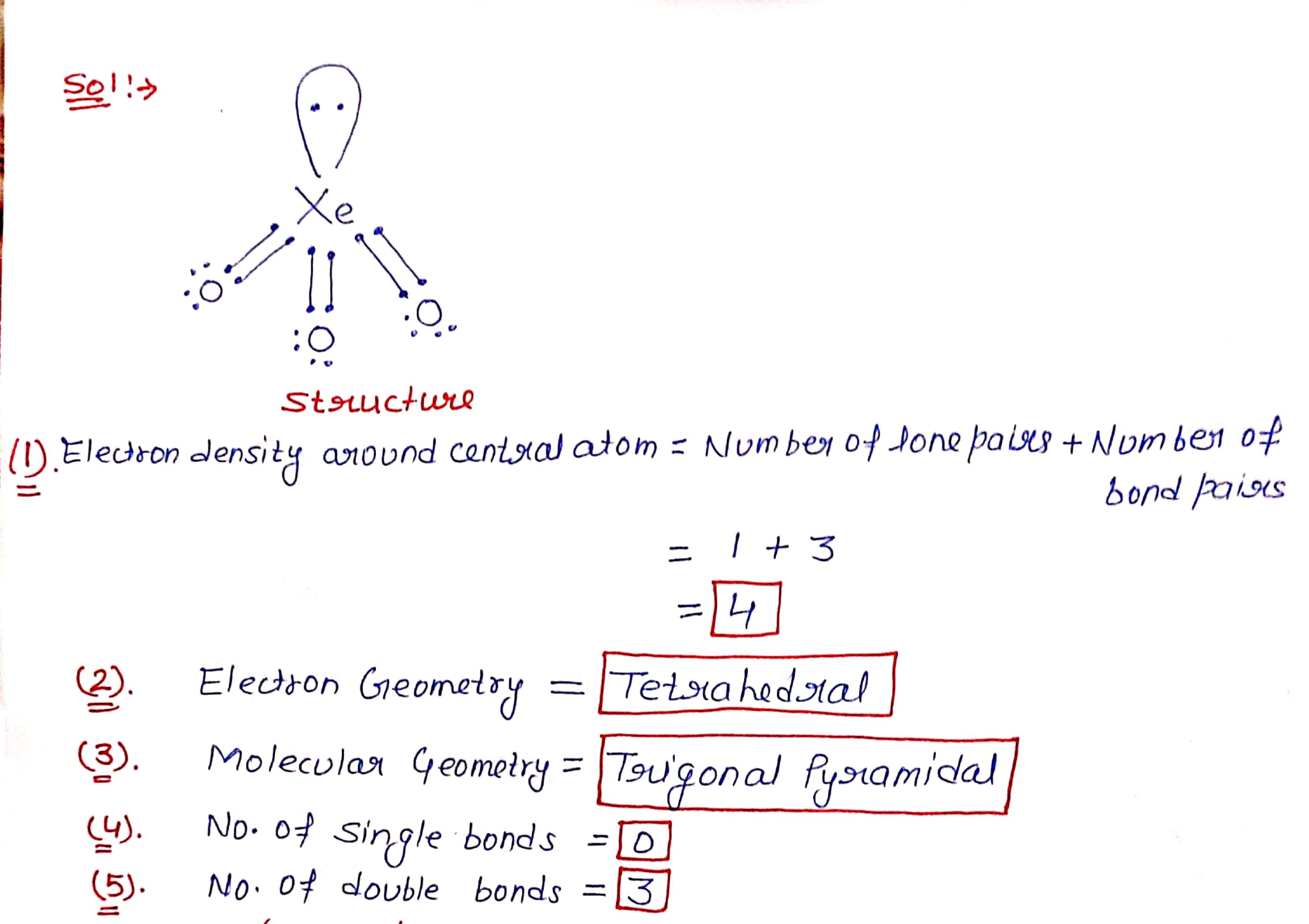#### Earn Coins

Coins can be redeemed for fabulous gifts.

Similar Homework Help Questions
• ### Using VSEPR theory, a molecule with the general formula AX E# (A is the central atom,...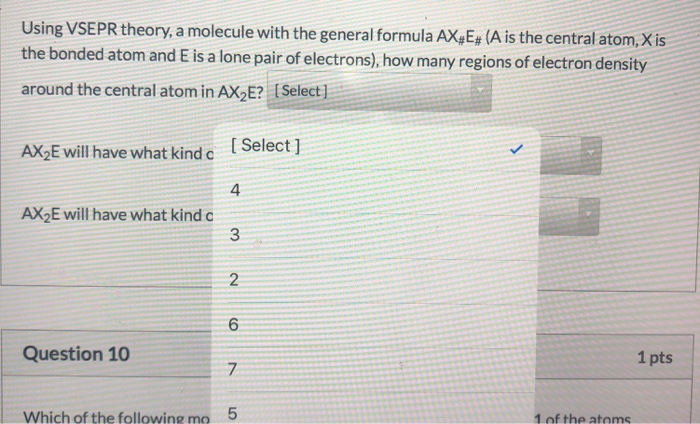Using VSEPR theory, a molecule with the general formula AX E# (A is the central atom, Xis the bonded atom and E is a lone pair of electrons), how many regions of electron density around the central atom in AX E? [Select] AX2E will have what kind c [Select] AX2E will have what kind a w N o Question 10 1 pts V Which of the following mo 5 1 of the atoms 5:35 PM Using VSEPR theory, a molecule...

• ### 10.) Specify the electron-domain geometry for the following two species using the VSEPR model: Xe...

10.) Specify the electron-domain geometry for the following two species using the VSEPR model: XeCl4 and IO4−. For each species, predict the bond angles based on the electron-domain geometry. (a)    XeCl4 geometry: linear trigonal planar    bent tetrahedral trigonal pyramidal trigonal bipyramidal seesaw T-shaped octahedral square pyramidal square planar pentagonal bipyramidal pentagonal pyramidal pentagonal planar bond angle: 60° 72°   90° 109.5° 120° 180° (b)    IO4− geometry: linear trigonal planar    bent tetrahedral trigonal pyramidal trigonal bipyramidal seesaw T-shaped octahedral square pyramidal square...

• ### Specify the electron-domain geometry for the following two species using the VSEPR model: KrCl4 and BrO4....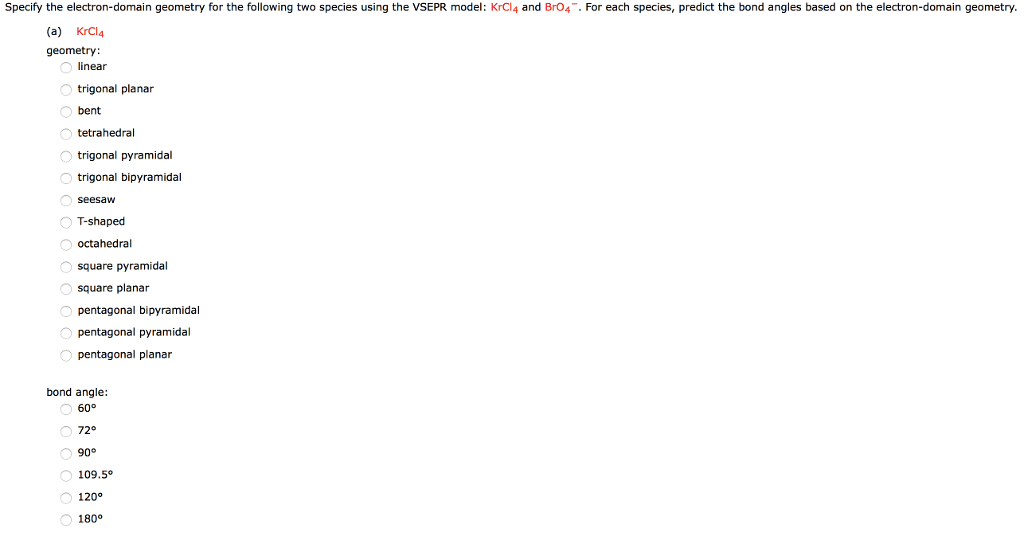Specify the electron-domain geometry for the following two species using the VSEPR model: KrCl4 and BrO4. For each species, predict the bond angles based on the electron-domain geometry. (a) KrCl4 geometry linear trigonal planar bent tetrahedral trigonal pyramidal trigonal bipyramidal T-shaped octahedral square pyramidal square planar pentagonal bipyramidal pentagonal pyramidal pentagonal planar bond angle: 72° 109.5° 120 180° (b) Br04 geometry: inear trigonal planar bent tetrahedral trigonal pyramidal trigonal bipyramidal seesaW T-shaped octahedral square pyramidal square planar pentagonal bipyramidal pentagonal...

• ### Draw the Lewis structure for PCl₆-, then answer the following questions:

Draw the Lewis structure for PCl₆-, then answer the following questions: A. How many valence electrons does this compound have? B. How many bonded electrons does this compound have? C. How many lone pairs of electrons does this compound have? D. How many single bonds does this compound have? E. How many double bonds does this compound have? F. What is the electron group arrangement {aka electron pair geometry} (enter one of the following: linear, trigonal planar, tetrahedral, trigonal bipyramidal, octahedral)? G. What is the molecular geometry {aka...

• ### VSEPR theory predicts which shape for the molecular structure of the following molecules or molecular ions?...

VSEPR theory predicts which shape for the molecular structure of the following molecules or molecular ions? (i.e. the arrangement of only the bonded atoms about the central atom) bent (or angular) linear octahedral see-saw square planar square pyramidal tetrahedral trigonal bipyramidal trigonal planar trigonal pyramidal T-shaped  ICl3 molecule    (The central atom is I.) bent (or angular) linear octahedral see-saw square planar square pyramidal tetrahedral trigonal bipyramidal trigonal planar trigonal pyramidal T-shaped  IO4- ion    (The central atom is I.) bent (or angular) linear...

• ### Draw the Lewis structure for AsH₃, then answer the following questions:

Draw the Lewis structure for AsH₃, then answer the following questions: A. How many valence electrons does this compound have? B. How many bonded electrons does this compound have? C. How many lone pairs of electrons does this compound have? D. How many single bonds does this compound have? E. How many double bonds does this compound have? F. What is the electron group arrangement {aka electron pair geometry}(enter one of the following: linear, trigonal planar, tetrahedral, trigonal bipyramidal, octahedral)? G. What is the molecular geometry {aka...

• ### olecular Geometry Saved Help Save & E Ch. Ex. 6 - Predict the Geometry Using VSEPR...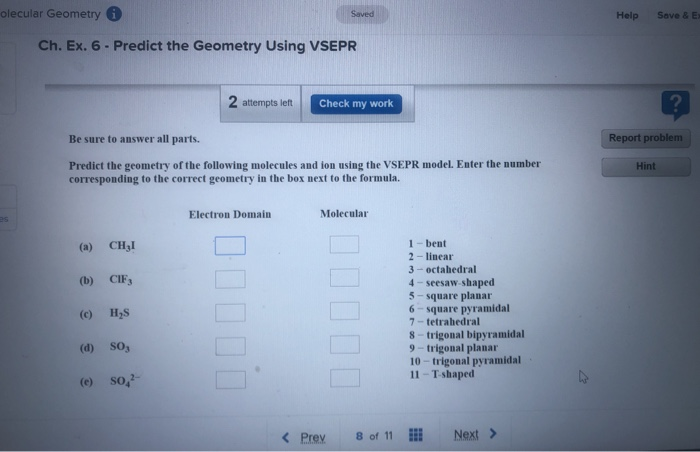olecular Geometry Saved Help Save & E Ch. Ex. 6 - Predict the Geometry Using VSEPR 2 attempts left Check my work Be sure to answer all parts. Report problem Hint Predict the geometry of the following molecules and ion using the VSEPR model. Enter the number corresponding to the correct geometry in the box next to the formula Electron Domain Molecular CHI 1 - bent 2 - linear 3-octahedral 4 - seesaw-shaped 5-square planar 6 - square pyramidal 7-tetrahedral...

• ### Provide the correct molecular geometry for (c), given the number of lone pairs and bonding groups...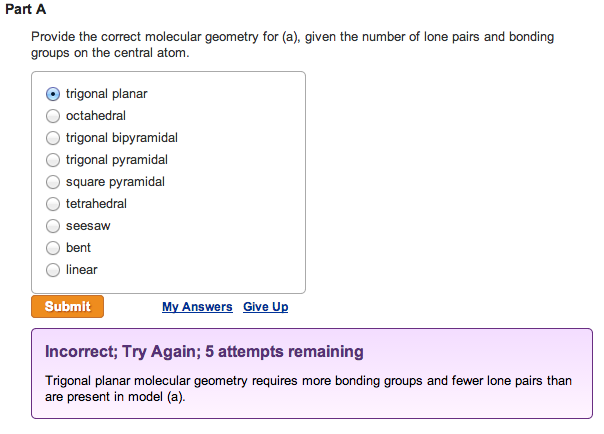Provide the correct molecular geometry for (a), given the number of lone pairs and bonding groups on the central atom. trigonal planar  octahedral  trigonal bipyramidal  trigonal pyramidal  square pyramidal  tetrahedral  seesaw  bent  linearProvide the correct molecular geometry for (b), given the number of lone pairs and bonding groups on the central atom.  trigonal bipyramidal  square pyramidal  bent  tetrahedral  trigonal planar  seesaw  trigonal pyramidal  octahedral  linear Provide the correct molecular geometry for (c), given the number of lone pairs and bonding groups on the...

• ### From the given Lewis structure and what you know about VSEPR theory, identify the shape of the molecule. х- -X trigonal...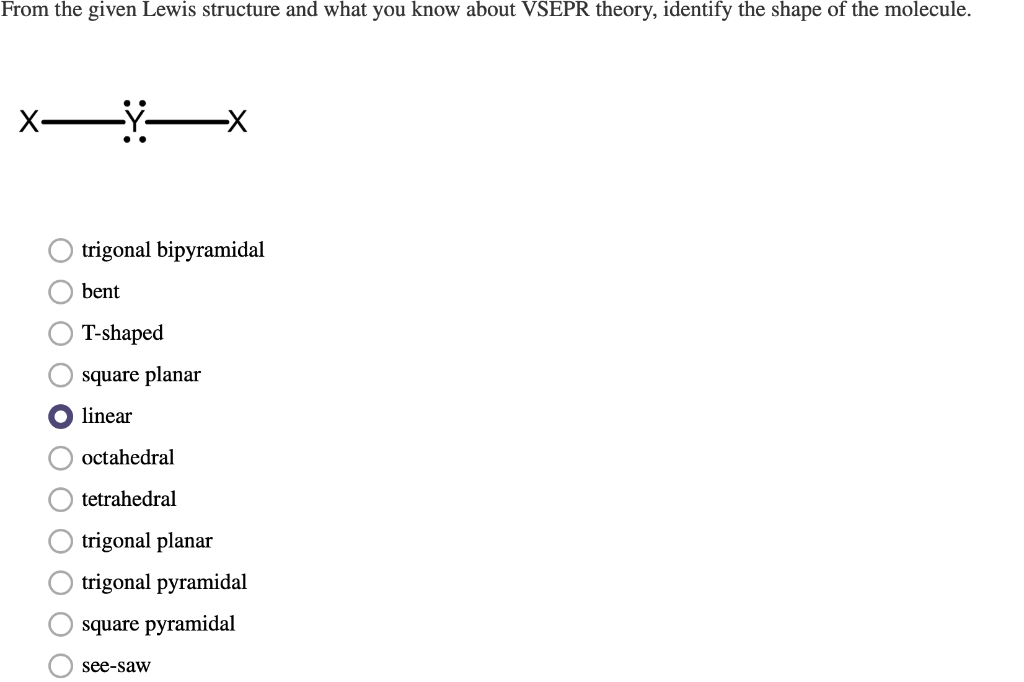From the given Lewis structure and what you know about VSEPR theory, identify the shape of the molecule. х- -X trigonal bipyramidal bent T-shaped square planar linear octahedral tetrahedral trigonal planar trigonal pyramidal square pyramidal see-saw OOO O O O O

• ### Determine the electron geometry and molecular geometry of KrF2 according to VSEPR theory. electron geometry =...

Determine the electron geometry and molecular geometry of KrF2 according to VSEPR theory. electron geometry = tetrahedral and molecular geometry = bent electron geometry = linear and molecular geometry = linear electron geometry = octahedral and molecular geometry = linear electron geometry = trigonal bipyramidal and molecular geometry = linear electron geometry = trigonal bipyramidal and molecular geometry = bent